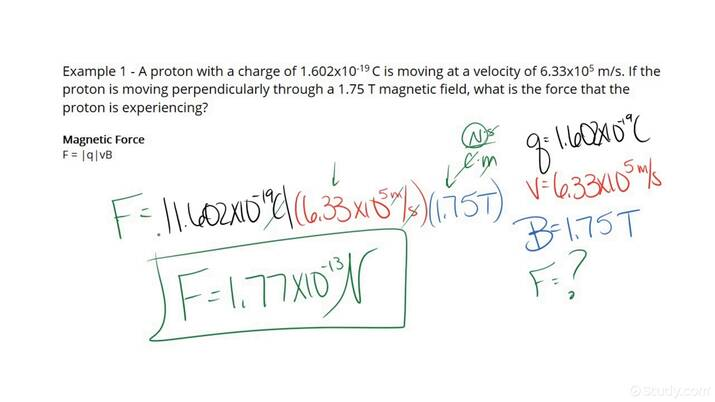# How to Calculate the Magnetic Force Perpendicular to the Direction of a ChargeAn error occurred trying to load this video.

Try refreshing the page, or contact customer support.

• 00:04 How to Calculate the…
• 02:27 How to Calculate the…
Maria El-Amin, Kirsten Wordeman
• Instructors
Maria El-Amin

Maria E. has a Bachelor’s in Physics form the University of Athens and Master’s and PhD degrees in Physics from the University of Toledo. She has 11 years of teaching experience in higher education, and 5 years of experience as a High-school principal. She has also tutored extensively.

•
Kirsten Wordeman

Kirsten has taught high school biology, chemistry, physics, and genetics/biotechnology for three years. She has a Bachelor's in Biochemistry from The University of Mount Union and a Master's in Biochemistry from The Ohio State University. She holds teaching certificates in biology and chemistry.

## How to Calculate the Magnetic Force Perpendicular to the Direction of a Charge

Step 1: Read the problem and identify the values of the charge q, the value of the velocity of the particle v and the value of the magnetic field B.

Step 2: Put these values into the equation: {eq}\rm{F=|q|vB} {/eq}.

Note: |q| is the absolute value of the charge q.

Step 3: Using this equation calculate the force F.

## What is the magnetic force?

Just like a charge that does not move produces an electric field, a moving charge produces a magnetic field. An object inside a magnetic field experiences a force that we call magnetic force.

Force: A push or a pull that can change an object's motion. The standard unit for force is a Newton (N).

Charge: The property of an object that allows it to be affected inside an electric or magnetic field. The standard unit of charge (also referred to as electric charge) is 1 Coulomb.

Velocity: The distance that an object travels in a unit of time. The velocity of an object includes the direction that the object travels to. The standard unit of velocity is m/s.

Magnetic field: An area in space where magnetic forces exist. We can visualize a magnetic field when, for example, we place iron fillings on a piece of paper and a magnet underneath the paper. The standard unit of the magnetic field is 1 Tesla. {eq}\rm{\left(T=\frac{N \, s}{C \, m }\right)} {/eq}

We will now solve two problems (step-by-step) to enforce our understanding as to how to calculate the magnetic force perpendicular to the direction of a charge.

## Examples of How to Calculate the Magnetic Force Perpendicular to the Direction of a Charge

Example 1

A proton is moving perpendicularly at {eq}\rm{4.5 \times 10^{6} m/s} {/eq} through a magnetic field of 1.5 T. What is the magnetic force that the proton experiences? Charge of a proton: {eq}\rm{1.602 \times 10^{-19} C} {/eq}

Step 1: Read the problem and identify the values of the charge q, the value of the velocity of the particle v and the value of the magnetic field B.

Charge: {eq}\rm{1.602 \times 10^{-19} C} {/eq}

Velocity: {eq}\rm{4.5 \times 10^{6} m/s} {/eq}

Magnetic field: Magnetic field: 1.5 T

Step 2: Put these values into the equation: {eq}\rm{F=|q|vB} {/eq}.

{eq}\rm{F=|1.602 \times 10^{-19} C|(4.5 \times 10^{6} m/s)(1.5 T)} {/eq}

Step 3: Using this equation calculate the force F.

{eq}\rm{F=|1.602 \times 10^{-19} C|(4.5 \times 10^{6} m/s)(1.5 T)} {/eq}

Since {eq}\rm{T=\frac{N \, s}{C \, m }} {/eq} all the units cancel except the Newtons:

{eq}\rm{F=1.1 \times 10^{-12} N} {/eq}

Our units are correct.

{eq}\rm{F=1.1 \times 10^{-12} N} {/eq}

Example 2

A charged particle carrying a charge of -2.5 C is moving perpendicularly to the magnetic field of the earth with a speed of 300,000 m/s. What is the force that the particle experiences if the magnetic field of the earth is 0.00003 T?

Step 1: Read the problem and identify the values of the charge q, the value of the velocity of the particle v and the value of the magnetic field B.

Charge: -2.5 C

Velocity: 300,000 m/s

Magnetic field: Magnetic field: 0.00003 T

Step 2: Put these values into the equation: {eq}\rm{F=|q|vB} {/eq}.

{eq}\rm{F=|-2.5 C| (300,000 m/s)(0.00003 T)} {/eq}

Step 3: Using this equation calculate the force F.

{eq}\rm{F=|-2.5 C| (300,000 m/s)(0.00003 T)} {/eq}

Since {eq}\rm{T=\frac{N \, s}{C \, m }} {/eq} all the units cancel except the Newtons:

{eq}\rm{F= 20 N} {/eq}